## 2-Step Word Problems Involving the Four Operations

#### 1. Mr. Baker bought a guitar and a microphone. The guitar cost \$1589 and the microphone cost \$572. If he had \$3500 at first, how much money had he left after paying for the two items?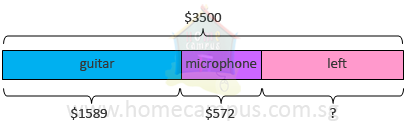\$1589  +  \$572  =  \$2161
The guitar and the microphone cost \$2161 altogether.

\$3500  −  \$2161  =  \$1339
He had \$1339 left after paying for the two items.

#### 2. 2447 children participated in a drawing competition. 1250 of them were boys. How many fewer girls than boys participated in the competition?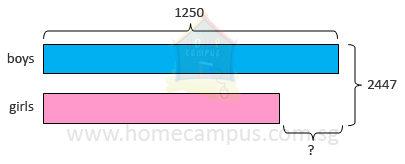2447  −  1250  =  1197
There were 1197 girls.

1250  −  1197  =  53
53 fewer girls than boys participated in the competition.

#### 3. Bakery A sold 2088 muffins. Bakery B sold 621 more muffins than Bakery A. What is the total number of muffins sold by the two bakeries?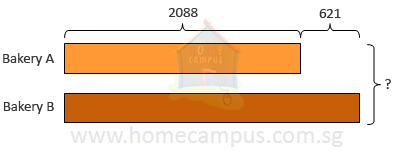2088  +  621  =  2709
Bakery B sold 2709 muffins.

2088  +  2709  =  4797
The total number of muffins sold by the two bakeries is 4797.

#### 4. Mr. Cooper made 8 boxes of cheese rolls. Each box contained 165 cheese rolls. He kept 212 for his family and sold the rest. How many cheese rolls did he sell?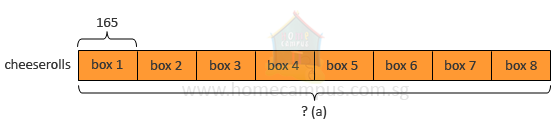165  ×  8  =  1320     (a)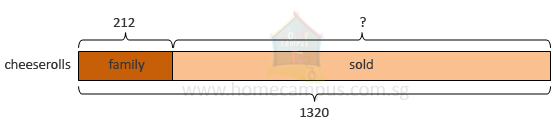1320  −  212  =  1108
He sold 1108 cheese rolls.

#### 5. Julie had some pencils and erasers. She put 3 pencils and 5 erasers into each pencil case. She got a total of 9 pencil cases.How many pencils and erasers were there altogether?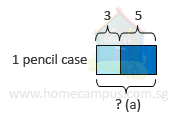3  +  5  =  8     (a)
There were 8 pencils and erasers in each pencil case.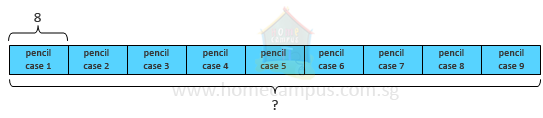8  ×  9  =  72
In 9 pencil cases, there were 72 pencils and erasers altogether.

#### 6. James, Kai and Lee liked collecting stickers. Lee had 6 times as many stickers as Kai. James had 94 more stickers than Lee. Kai had 193 stickers. How many stickers did James have?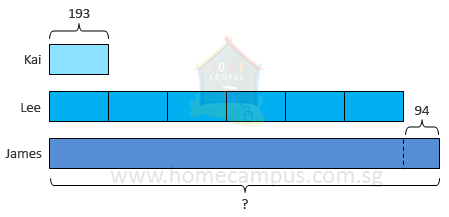193  ×  6  =  1158

1158  +  94  =  1252

#### 7. A bag of cake mix costs \$15. Aryanna had \$127 left after buying 5 bags of cake mix. Find the sum of money she had at first.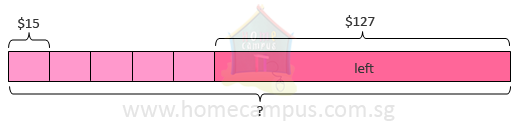\$15  ×  5  =  \$75
The 5 bags of cake mix cost \$75 altogether.

\$75  +  \$127  =  \$202

#### 8. Mr. Roberts had 540 bananas. He separated 76 ripe bananas. He sold the remaining bananas equally to 8 fruit stalls. How many bananas did each fruit stall get?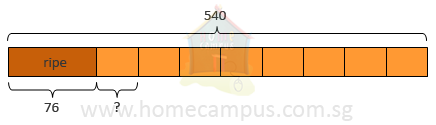540  −  76  =  464
He sold 464 bananas altogether.

464  ÷  8  =  58
Each fruit stall got 58 bananas.

#### 9. Billy bought 5 bags of balls. Each bag contained 40 balls. He packed the balls into packets of 8 balls each. How many packets did he get?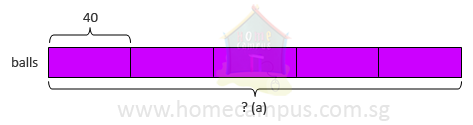40  ×  5  =  200     (a)
There were 200 balls altogether.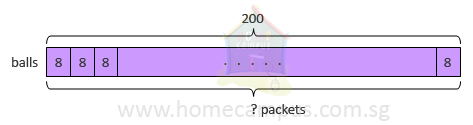200  ÷  8  =  25
He got 25 packets.

#### 10. A boy collects a total of 316 stamps, stickers and coins. He collects four times as many stamps as stickers. There are 46 coins. How many stickers are there?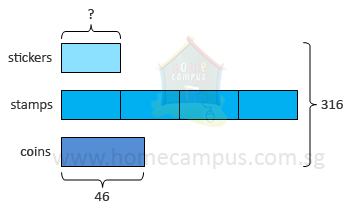316  −  46  =  270
There are 270 stamps and stickers.

270  ÷  5  =  54
There are 54 stickers.

#### 11. There were a total of 19 chickens and goats on a farm. Adam counted 54 legs altogether. How many goats were there on the farm? [Hint: A chicken has 2 legs. A goat has 4 legs.]Start by counting 2 legs on each creature - chicken as well as goat - as each of them has at least 2 legs.

19  ×  2  =  38
So far, we have counted 38 legs, which include both the legs on each chicken and 2 legs on each goat.

4  −  2  =  2
For each goat, we need to count 2 more legs.
54  −  38  =  16
16 legs remain to be counted.

The remaining 16 legs belong to the goats; 2 for each goat.
16  ÷  2  =  8
There were 8 goats.